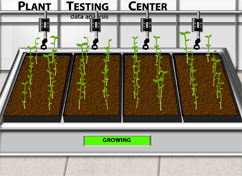# Data AnalysisHow many times in science class have you collected some data, calculated some averages, and then created a conclusion based on those calculations? If you are like most students, you have done or will do this many times in your educational career. However, there is a big problem with this approach. You didn’t do an additional step to find out if your results were significant or not. Hopefully, you’re ready to take the next step in becoming a scientist: analyzing your results.

### What are variables?

A variable is a factor that can be manipulated or measured. The variable that is manipulated is called the independent variable, and the variable that is measured is called the dependent variable.

### What is an operational definition?

The operational definition states how the variables will be measured in a given experiment. For instance, the dependent variable growth could be measured in a variety of ways, some good and some less valuable. One way to measure growth would be to count the number of fruit a tree produced. Another operational definition for growth could be the increase in height of a plant. A given experiment must always define how the variables will be measured.

### What is a hypothesis?

A good third-grade definition of a hypothesis is "an educated guess." However, a much better definition exists that more closely aligns with scientific thinking. This defines a hypothesis as a "prediction based on prior information of what may occur that can be experimentally tested." A good form to write your hypothesis is, "the independent variable affects the dependent variable."

### What is the sample size?

The sample size is the total number of subjects or things in a study. For instance, if a study has two groups, with ten people in each group, the sample size would be 20. The accepted number most scientists generally use for the minimum sample size is 30. However, a larger sample size usually provides better experimental results.

### What is the difference between a control and experimental group?

In the control group, no experimental conditions are applied. This means that the control group is not manipulated in any way so that it can be used as a reference. The independent variable is applied to the experimental group so that its effect can be measured.

### What is the mean (average)?

The mean, or average, of a data set is a number that represents the general value of the data set. The mean is computed by adding up all the data and dividing this sum by the number of data. For instance, if you had five students who took a test and you wanted to compute the mean score, you would add up all five test scores and then divide this number by five.

### What is a Student's t-test?

A Student's t-test is a statistical test used to determine if a real, significant difference exists between the averages of two groups. The averages of two groups could be numerically different, yet those differences in values could be due to randomness in the data and not due to a cause-effect relationship between the independent and dependent variables. The t-test determines whether the differences in the averages are real or not. When a t-test is calculated, a "p" value is determined. If the "p" value is less than 0.05, then a real difference exists between the group's averages and one variable does affect the other one. If the "p" value is greater than 0.05, then no real difference exists between the group's averages and one variable does not affect the other one.